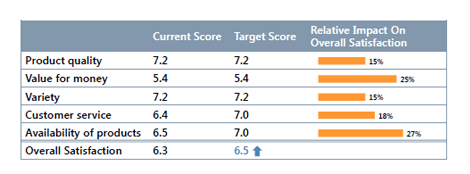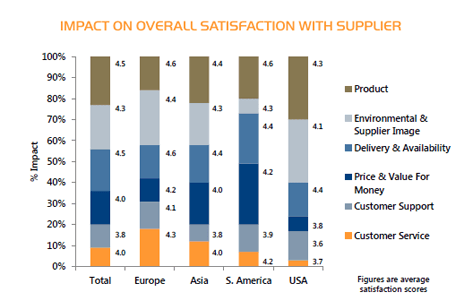From overall customer satisfaction to satisfaction with your product quality and price, regression analysis measures the strength of a relationship between different variables.

### How regression analysis works

While correlation analysis provides a single numeric summary of a relation (“the correlation coefficient”), regression analysis results in a prediction equation, describing the relationship between the variables. If the relationship is strong – expressed by the Rsquare value – it can be used to predict values of one variable given the other variables have known values. For example, how will the overall satisfaction score change if satisfaction with product quality goes up from 6 to 7?### Measuring customer satisfaction

Regression analysis can be used in customer satisfaction and employee satisfaction studies to answer questions such as: “Which product dimensions contribute most to someone’s overall satisfaction or loyalty to the brand?” This is often referred to as Key Drivers Analysis.

It can also be used to simulate the outcome when actions are taken. For example: “What will happen to the satisfaction score when product availability is improved?”